## Introduction

Low energy band structure in graphene is formed by two spin-degenerate massless Dirac cones at two inequivalent valleys, giving rise to four massless Dirac cones in total1,2. With one additional graphene layer added, bilayer graphene (BG) has an entirely different band structure. Most notably, symmetric BG is a zero-band gap semiconductor with quadratic energy-momentum dispersion3. However, its band gap is continuously tunable through an electrical field applied perpendicularly to the sample4,5. The electronic states in BG are also four-fold degenerate, taking into account both spin and valley degeneracies. Recently, it has been shown that HgTe quantum wells (QWs) with vanishing band gap possess a single spin-degenerate Dirac cone at the Brillouin zone center6,7,8,9 and thus can be considered as the “single-valley” analog of graphene in two-dimensional (2D) semiconductor heterostructures.

The central feature of the HgTe QWs is the possibility of band inversion. The barrier material (e.g., CdTe) has a normal band ordering, with the s-type Γ6 band lying above the p-type Γ8 band, while in the well material (HgTe) the Γ6 band lies below the Γ8 band, corresponding to an inverted band ordering. If the thickness d of the HgTe/CdTe QWs does not exceed a critical value dc, at the Γ point, the first conduction subband (E1) is electron-like, i.e. it is formed by a linear combination of the |Γ6, mJ = ±1/2〉 and |Γ8, mJ = ±1/2〉 states, while the first valence subband (H1) arises from the |Γ8, mJ = ±3/2〉 states, corresponding to the heavy-hole band. In wide HgTe QWs (d > dc), the E1 subband falls below the H1 subband. The inversion between E1 and H1 subbands leads to the formation of a 2D time-reversal invariant topological insulator (TI)10 denoted the quantum spin Hall (QSH) state6,7 with dissipation less edge channel transport at zero external magnetic field11. At critical QW thickness dc, corresponding to topological phase transition between a TI and a trivial band insulator (BI), a low-energy band structure mimics a massless Dirac cone at the Γ point6.

Two tunnel-coupled HgTe QWs of critical thickness may, therefore, share some properties of natural BG. If each of the HgTe QW has d > dc, this system offers a practical realization of tunnel-coupled layers of 2D TI. Moreover, the strained HgTe thick films have been proven to be three-dimensional (3D) TIs12,13. Therefore, a wide double HgTe QW can be considered as two thick layers of 3D TI separated by BI (CdTe material) with the surface states arising at the interfaces14. A tensile strain in the HgTe films, which opens a topological gap, is induced by the difference in the lattice parameters of HgTe and CdTe. Thus, double HgTe QWs is a realistic system, which potentially paves the way towards physics of multi-layered topological insulator materials.

So far, there are just a few works devoted to double HgTe QWs15,16,17. All these works are based on the approach, in which each QW is described within Bernevig-Hughes-Zhang (BHZ) model6 coupled by a spin-conserved tunneling Hamiltonian. The latter will be shown to be irrelevant on double HgTe QWs, which feature a much richer physics than previously assumed.

In our theoretical investigation of double HgTe QWs (see Fig. 1), we first start from band structure calculations on the basis of an eight-band Kane model18. By using realistic material parameters, we obtain the phase diagram for symmetrical double QW as a function of the layer thicknesses. We discover a specific metal phase, in which the band properties in perpendicular magnetic and electric fields are very similar to the ones of natural BG. Then, we deduce an effective 2D Hamiltonian, involving two electron-like (E1, E2) and two hole-like (H1, H2) subbands, to describe quantum phase transitions in the system. On the basis of such a simplified Hamiltonian, we calculate dispersion of edge states in different phases. We show that inversion between E2 and H2 subbands induces an additional pair of helical edge states, putting the system into BI phase even in the case of inverted band structure. The crossing between E2 and H2 levels yields a Dirac cone in the Γ point of the Brillouin zone.

Considering double HgTe QW, shown in Fig. 1, as a whole system and by using expansion in the plane-wave basis set (see Supplementary Information), we calculate energy dispersions of electronic subbands. Figure 2A,B show positions of electron-like and hole-like subbands at k = 0 as a function of middle barrier thickness t. Intuitively, it is clear that at infinitely large barrier, calculated states correspond to the subband positions in two separated HgTe QWs, while at finite values of t each pair of the subbands is connected with even-odd state splitting in double QWs. If t tends to zero, the energy values transform into positions of electronic subbands in single HgTe QWs of 2d thickness. It is seen that the splitting between electron-like levels exceeds significantly the one of the hole-like levels. This is due to significant difference in effective masses at k = 0 in electron-like and hole-like subbands, which values determine the tunnel-coupling between the states and their even-odd splitting at given t.

There are two types of the band structure ordering, which arises depending on the HgTe layers thickness d. The first case shown in Fig. 2A takes place if d varies in the range from dc/2 to dc, where dc corresponds to the thickness of single QW with Dirac cone at the Γ point. In this case E2 subband always lies above H2 subband and inversion between E1 and H1 subband can take place. At higher values of t, the E1 subband gains a higher energy than the H1 subband and the system has the normal band structure. When the thickness t is decreased, the energy of the E1 subband reduces, whereas the H1 subband energy practically does not change. The different dependence of E1 and H1 subbands on t implies that at some barrier thicknesses the band gap closes. In fact, the crossing point between E1 and H1 subbands yields a low-energy band structure with Dirac cone in the vicinity of k = 0 (Supplementary Information). If the values of t are small enough to induce a gap between H1 and H2 subbands, it implies a quantum phase transition between BI and TI states, as in single HgTe QW6. However, if H1 and H2 subbands coincide at k = 0, it gives rise to an additional “massive” branch of valence subband at the crossing point, similar to the pseudospin-1 Dirac-Weyl 2D systems19, as well as a quantum phase transition into a specific metal phase with the following subband ordering: E2-H1-H2-E1. The reasons to assign this metal phase as a BG phase are discussed later.

The second type of band structure ordering, shown in Fig. 2B, is realized when d > dc. In this case E1 subband always lies below H1 subband, i.e. they are inverted. At lower barrier thickness, E2 subband lies above H2 subband, while at high values of t, they swap their positions. At specific values of t, E2 and H2 subbands cross, which also leads to the appearance of a Dirac cone at the Γ point. As for the case shown in Fig. 2A, additional “massive” branch arises as well. However, such branch corresponds to conduction H1 subband. Up to date, it was never realized that crossing between E2 and H2 subbands also induces a quantum phase transition. In this work we explicitly demonstrate it for the first time. In particular, we show that this crossing point in double HgTe QWs correspond to the quantum phase transition between BG and BI phase.

Figure 2C shows the phase diagram, in which two bold lines correspond to the Dirac cones at the Γ point. The left one results from the crossing between E1 and H1 subbands, while the right-side curve is connected with the crossing of E2 and H2 levels. If the middle barrier is thin enough, a gap between H1 and H2 subbands opens and the inversion between E1 and H1 levels induces a quantum phase transition between BI and TI phases. The latter is shown by orange region. For relatively wide QWs, the so-called semimetal (SM) phase, corresponding to the white-striped region, is implemented. It is characterized by a vanishing indirect band gap, when the side maximum of the valence subband exceeds in energy the conduction subband bottom. This phase arises in single HgTe QWs as well when the thickness goes beyond some critical value, denoted in Fig. 2C by dSM20,21. BG metal phase mentioned above corresponds to the blue region in Fig. 2C. Dispersion curves at various values of d and t in the vicinity of phase transitions between BI, BG and SM phases are provided in Supplementary Information.

Let us now explain the reasons to call this specific metal phase with the ordering of electronic subbands E2-H1-H2-E1 a BG phase. First, we consider in details the case when both QWs have a critical thickness dc ≈ 6.5 nm, which at infinitely large barrier corresponds to two Dirac cones. The presence of the transparent barrier, for example of t = 3 nm, turns from two Dirac cones in the vicinity of the Γ point into a band structure very similar to the one of natural BG3 (c.f. Fig. 3A). In particular, it consists of two gapless isotropic parabolas, formed by H1 and H2 subbands. Moreover, as in BG in which non-zero band gap can be induced by breaking the inversion symmetry of two monolayers, in double HgTe QWs it could be obtained by using QWs of different thickness or by adding of one-side chemically doping. Moreover, the potential of a continuously tunable band gap through an electric field applied perpendicularly to the sample plane is of particular importance.

The double HgTe QW in BG phase also holds this property. Figure 3B displays energy dispersion for BG phase in perpendicular electric field of 20 kV/cm. Even in this case the dispersion curves are very similar to the band structure of natural BG in external electric field3,4. However, strong spin-orbit interaction in HgTe layers removes the spin degeneracy away from the Γ point due to Rashba effect22. Figure 3C shows the band gap values in the double QWs in BG phase as a function of applied electric field. Indeed, the band gap in BG phase is electrically-tunable, as it is in natural BG. However, its dependence on electric field has non-monotonic behaviour in double HgTe QWs. The reason for this is related with the additional side maximum of valence subband, which is also responsible for the formation of SM phase in zero electric field (see the diagram, shown in Fig. 2C). The top of the side maximum is increasing with the strength of electric field and the band gap reduces (see the inset, shown in Fig. 3C). As a result, the band gap is closed in high enough electric field, giving rise to the formation of the SM phase. A critical electric field, corresponding to arising of indirect-band gap, coincides with the maximum value of Δ, shown in Fig. 3C.

Another characteristic of natural BG is the unconventional quantum Hall effect, related with the absence of zero-Landau level (LL) plateaus in Hall conductivity23. For natural BG, plateaus in the Hall conductivity σxy, occur at integer multiples of 4e2/h. This is similar to a conventional semiconductor with level degeneracy g = 4 arising from the spin and valley degrees of freedom. Deviation from the conventional case occurs at low density, where there is a step in σxy of height 8e2/h across zero density, arising from the eightfold degeneracy of the zero-energy LL. This specific LL is formed by atomic orbitals of different sublattice sites from both layers24.

Figure 3D shows LL fan chart in double HgTe QW with BG phase, calculated within the eight-band Kane model. In the BG phase, in perpendicular magnetic field a specific zero-mode LL with degeneracy two times higher than other LLs also arises. This zero-mode LL is shown in Fig. 3D, by bold orange line. For double HgTe QW, plateaus in the Hall conductivity are expected to occur at integer numbers of e2/h. However, the doubled degeneracy of zero-mode LL requires twice as many carriers to fill them, so the transition between the corresponding plateaus should be twice as wide in density and the step in σxy between the plateaus are expected to be twice as high, 2e2/h instead of e2/h. Since the zero-mode LL in double HgTe QW is formed by the states of both H1 and H2 subbands, the presence of inversion asymmetry of two HgTe layers not only opens the band gap between H1 and H2 subbands (see Fig. 3B) but also splits the zero-mode LLs, removing the double degeneracy order. Therefore, we also expect recovering of sequence of equidistant plateaus in the Hall conductivity as it is for gate-biased natural BG5.

Besides the zero-mode LL, there are two additional specific LLs, which in high magnetic fields are formed only by the states from E1 and E2 subbands. These LLs are shown in Fig. 3D by blue curves. In moderate magnetic fields, these E1 and E2 LLs are mixed with the states from H2 and H1 subbands respectively. It results in anticrossing between E1 (E2) LL and LLs from H2 (H1) subband, which is clearly seen in magnetic fields below 1 T. The crossing between the E1 and zero-mode LL, arising at critical magnetic field Bc ~ 3.5 T, corresponds to the transition from inverted into normal band structure, similar to this observed in single HgTe QW7.

We have considered in details the case of double HgTe QW, when both HgTe layers have a critical thickness dc. However, all the mentioned properties of BG phase hold for any double QW with the values of d and t, corresponding to the blue region in Fig. 2C. The only difference is the ratio M1 over M2, where M1 parameter describes the energy gap between E1 and H1 subbands, while M2 corresponds to the half of the gap between E2 and H2 levels. If d < dc, 2M2 exceeds 2M1, while in the opposite case of d > dc, the gap between E2 and H2 subbands is lower than 2M1. An amazing property of double HgTe QW is that it shares some characteristics of natural BG even in the BI phase at d > dc. In particular, the possibility of tuning the band gap by electric field and of observing the unconventional double step in plateaus in the Hall conductivity still persists.

We now discuss quantum phase transitions in double HgTe QWs. For this purpose, we derive an effective 2D Hamiltonian, which describes the band structure in the vicinity of k = 0 in the phases shown in Fig. 2C. To infer this simplified model, we start from eight Bloch basic states, combined into an eight-component spinor: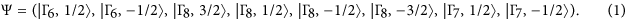For the QWs grown in  direction, projection mJ of total angular momentum at k = 0 on the growth direction is still a good quantum number. At the Γ point all QW subband states are formed by linear combination of the mentioned eight bulk bands. To describe BI, TI and BG phases, one should consider E1, E2, H1 and H2 subbands (see Fig. 2A,B). At k = 0, the |E1, ±〉 and |E2, ±〉 subband states are formed from the linear combination of the |Γ6, mJ = ±1/2〉, |Γ7, mJ = ±1/2〉 and |Γ8, mJ = ±1/2〉, while the |H1, ±〉 and |H2, ±〉 QW states are formed from the |Γ8, mJ = ±3/2〉 states. Away from the Γ point, the E1, E2, H1 and H2 states are mixed. To construct the effective Hamiltonian one should carefully take into account different parities of the envelope function components from |Γ6, ±1/2〉, |Γ7, ±1/2〉, |Γ8, ±1/2〉 and |Γ8, ±3/2〉 bulk bands into formation of given subband state25. For instance, E1 state is formed by even function corresponding to |Γ6, ±1/2〉 band and by odd envelope functions corresponding to |Γ7, ±1/2〉 and |Γ8, ±1/2〉 bands, while parities of their contributions into E2 state are changed. In previous works15,16,17, this point was missed; it results in the wrong description of double HgTe QWs. Detailed explanation is given in Supplementary Information.

After straightforward calculations, we obtain the following form of the effective 2D Hamiltonian for the E1, E2, H1, H2 states, expressed in the basis of two Kramer’s sets |E1, +〉, |H1, +〉, |H2, −〉, |E2, −〉 and |E2, +〉, |H2, +〉, |H1, −〉, |E1, −〉: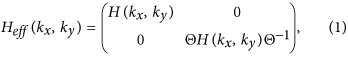where Θ is a “time reversal” operator, given by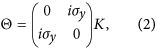with K stands for complex conjugation and σy is one of the Pauli spin matrices. Each block in (1) is described by a four-component spinor with pseudospin J = 3/2 degree of freedom (see Supplementary Information). H(kx, ky) is written as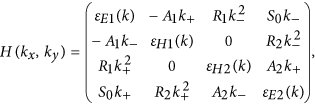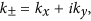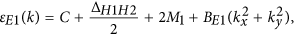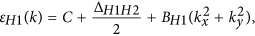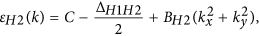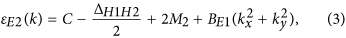Here, kx and ky are momentum components in the plane of double QW and C, M1, M2, A1, A2, BE1, BH1, BH2, BE2, ΔH1H2, R1, R2, S0 are specific heterostructure constants, being defined by QW geometry and materials. The Hamiltonian Heff (kx, ky) has block-diagonal form because we keep the inversion symmetry and axial symmetry around the growth direction (see Supplementary Information). We note that Heff (kx, ky) is valid for any values of d and t. In particular for t = 0, it describes energy dispersion in the vicinity of the Γ point in single HgTe QW beyond the BHZ model. Parameters ΔH1H2, R1, R2, S0 significantly depend on t and all tend to zero at large middle barrier thickness, while BE1 = BE2 coincides. As it is easy to see, in this case the system is described by two non-interacting BHZ models, written for two pairs formed by E1, H1 and E2, H2 subbands. We note that BH1 = BH2 if ΔH1H2 = 0.

The most important quantities in H(kx, ky) are two mass parameters M1 and M2. Almost all phases in Fig. 2C can be grouped into three types according to the sign of M1 and M2. The BI phase at normal band structure, shown in the left part of the diagram, corresponds to positive values of M1 and M2. The BG and TI phases arise if M1 < 0 and M2 > 0 and the difference between these phases is connected with ΔH1H2, which equals to zero in the case of BG phase. The second BI phase, shown in the right from the BG phase, corresponds to negative values of M1 and M2 and ΔH1H2 = 0. The SM phase, arising at all values of M2 and M1 > 0, cannot be described within the simplified model because it is only valid near the Γ point, while the SM phase is formed by non-local overlapping of the valence band and conduction band bottom20,21. Comparison between calculations of subband curves, performed within the simplified model and on the basic of an eight-band Kane Hamiltonian for different phases is given in Supplementary Information.

Zero values of M1 and M2 both conform to quantum phase transitions at which Dirac cone at the Γ point occurs. The left and right bold black curves in the phase diagram, shown in Fig. 2C, are related with M1 = 0 and M2 = 0 respectively. Figure 4 presents energy dispersion for the bulk and edge states in various phases in double HgTe QWs obtained within the simplified model. Dispersion of the bulk states are shown in black, while the orange and blue curves are the edge states, described by different blocks of Heff (kx, ky). The electrons in the edge states, marked by different colors, move in opposite directions. Figure 4 demonstrates that each change of sign of mass parameter M1 or M2 yields a pair of helical edge states, providing a quantum phase transition at M1 = 0 or M2 = 0. In particular, BG phase arising at M1 < 0 and M2 > 0 has a single pair of the edge states, coexisting with the gapless bulk states. If M1 < 0 and M2 = 0, the double HgTe QW mimics a 2D system with the presence of both bulk and edge massless fermions, which energy dispersions are shown in Fig. 4C.

Let us now remark the main difference between BG phase in double HgTe QW and natural BG. The electrons in natural BG are chiral particles with S = 1/2 pseudospin of freedom23,24 and pseudospin winding number of 225. It results from the commutation of operator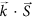with the low-energy Hamiltonian of natural BG. As it is shown above, the simplest Hamiltonian, required for the description of bulk and edge states in the BG phase, has J = 3/2 pseudospin of freedom. Moreover, it can be shown that operator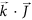does not commute with H(kx, ky), proving the non-chiral character of electron in double HgTe QWs. Thus, even though BG phase mimics some characteristics of natural BG, it is a novel and fascinating state of matter, exhibiting the coexistence of gapless bulk states and spin-polarized edge channels.